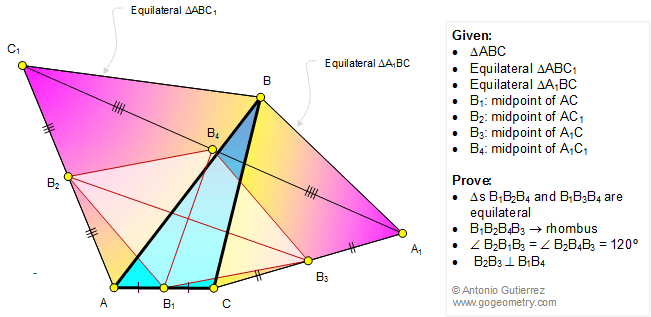# Online Math: Geometry Problem 1219: Equilateral Triangles, Midpoints, 60 Degrees, Congruence, Rhombus. Tiled background image: Intihuatana, Machu Picchu. Level: College, High School.

< PREVIOUS PROBLEM  |  NEXT PROBLEM >

 In the figure below, equilateral triangles ABC1 and A1BC are drawn on the sides of a triangle ABC. If B1, B2, B3, and B4 are the midpoints of AC, AC1, A1C, and A1C1, respectively, prove that (1) triangles B1B2B4 and B1B3B4 are equilateral; (2) B1B2B4B3 is a rhombus; (3) angle B2B1B3 = angle B2B4B3 = 120 degrees; (4) B2B3 and B1B4 are perpendicular.Home | SearchGeometry | Problems | All Problems | Open Problems | Visual Index | 10 Problems | Problems Art Gallery Art  | 1211-1220 | Triangles | Equilateral triangle | Midpoint | Congruence | 60 degrees | Rhombus | Email | by Antonio Gutierrez

Post or view a solution to the problem 1219
Last updated: May 29, 2016